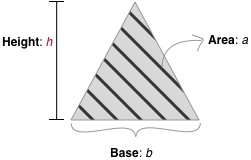Triangles

HackerRank: Minimum Height Triangle

It is always fun to work with triangles in any kind of setting not least problem solving in HackerRank. The problem Minimum Height Triangle is no exception. The problem reads

Given integers b  and a, find the smallest integer h, such that there exists a triangle of height h, base b, having an area of at least a.In case we should have forgotten the primary school mathematics. The area of a triangle is given by

This can be isolated such that we get the height of the triangle instead as

Since we want the minimum integer size we then need to ceil that such that we get

This can be implemented in Python as

def lowestTriangle(base, area):
return math.ceil(2 * area / base)

I usually write somewhat longer posts. But to be honest, I don’t know what to add to this problem.

Posted by Kristian in HackerRank, 0 comments